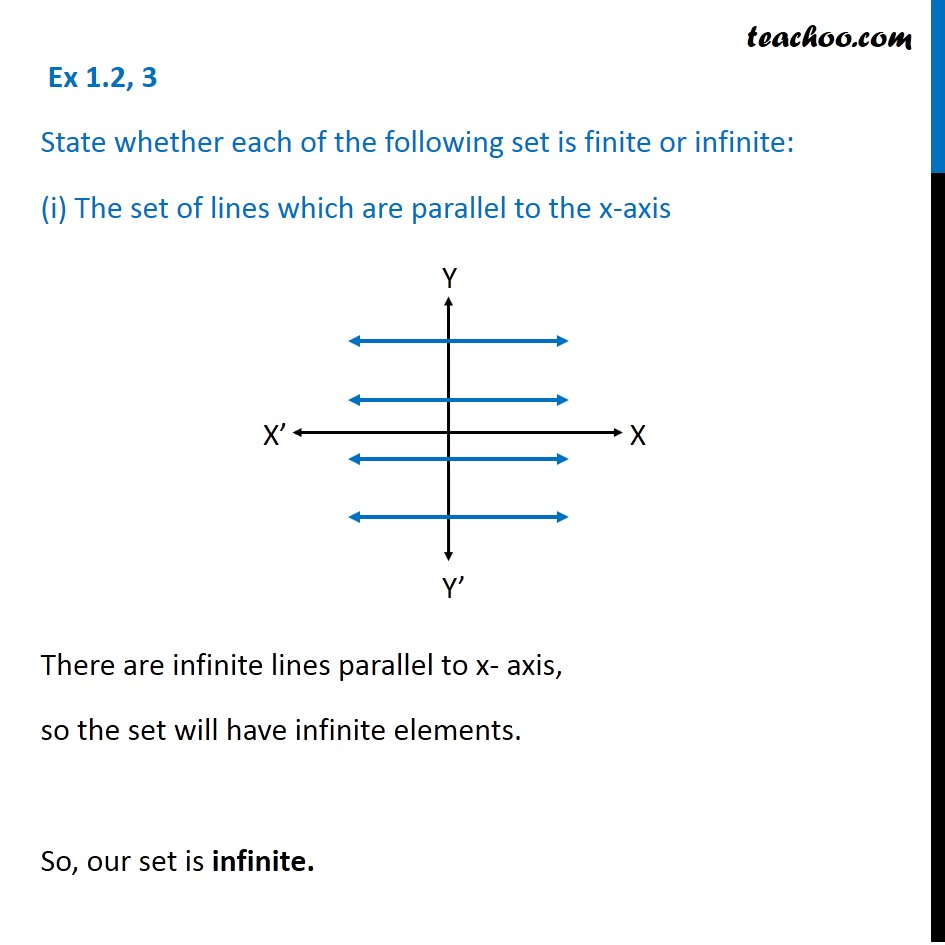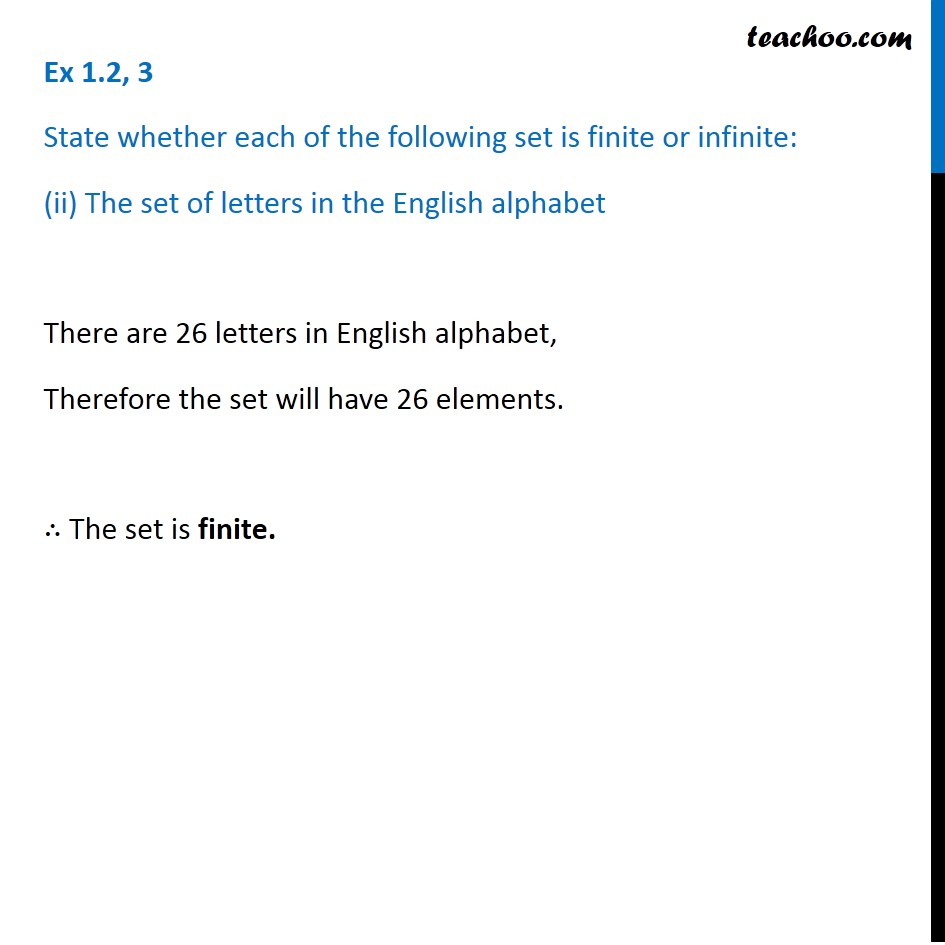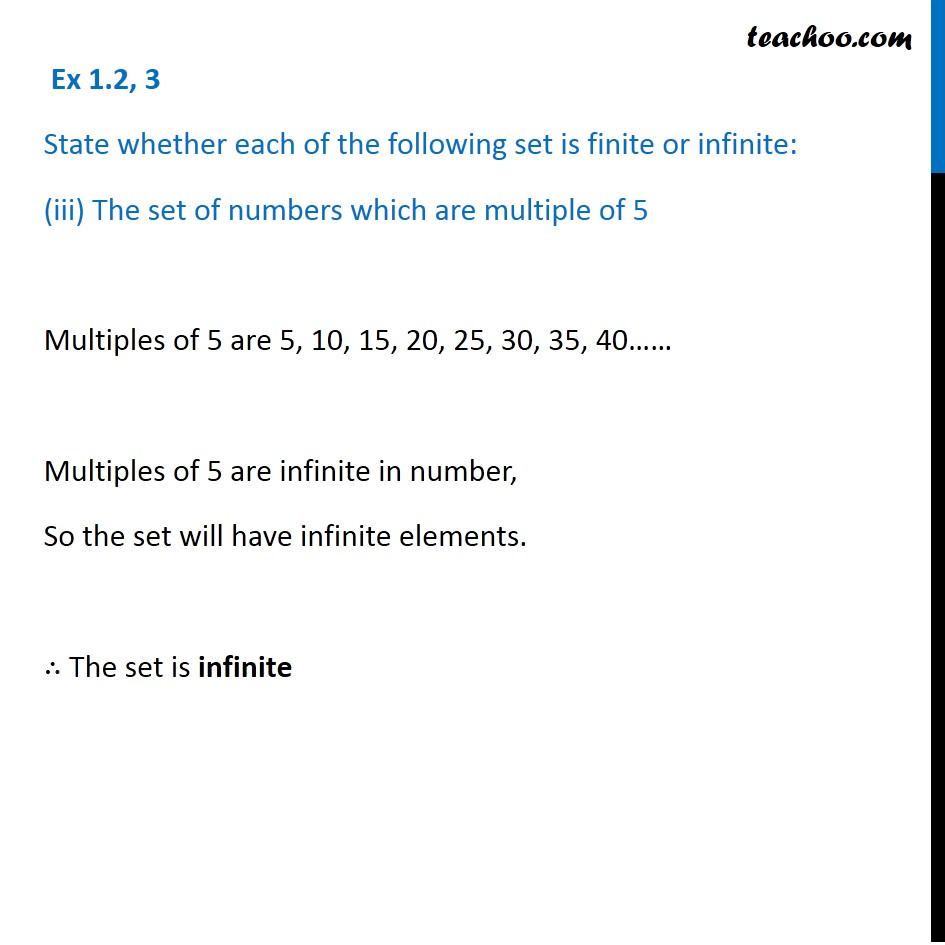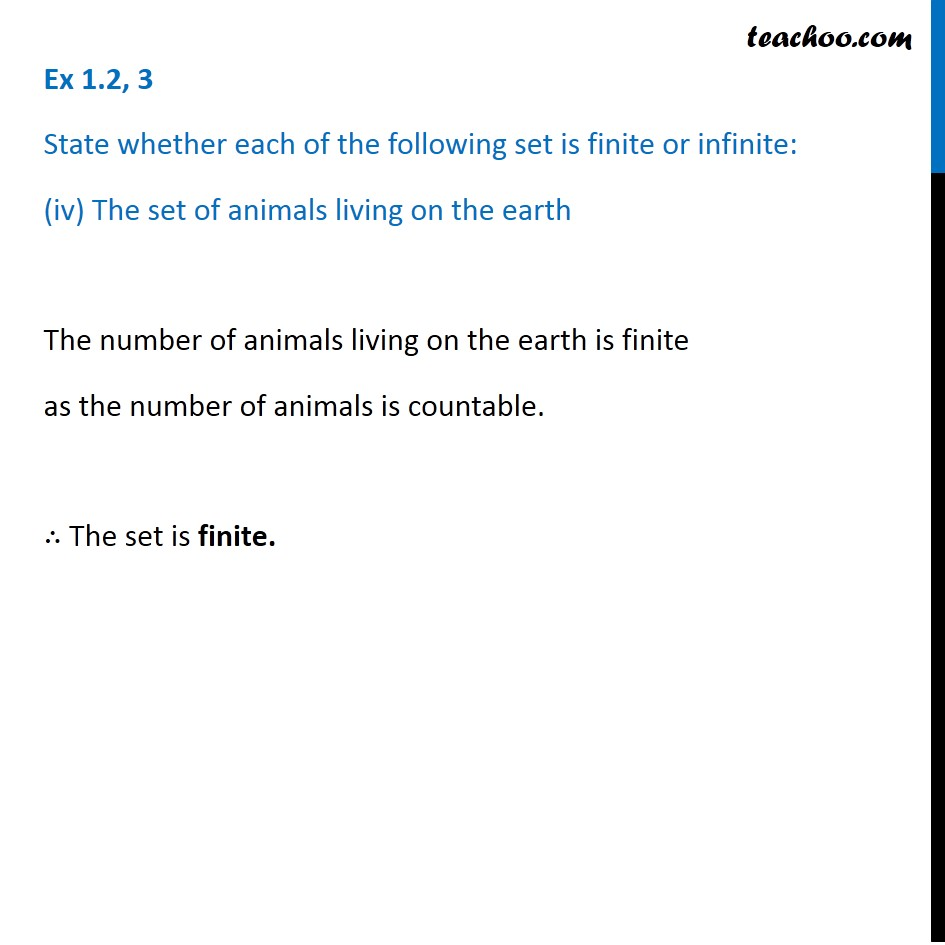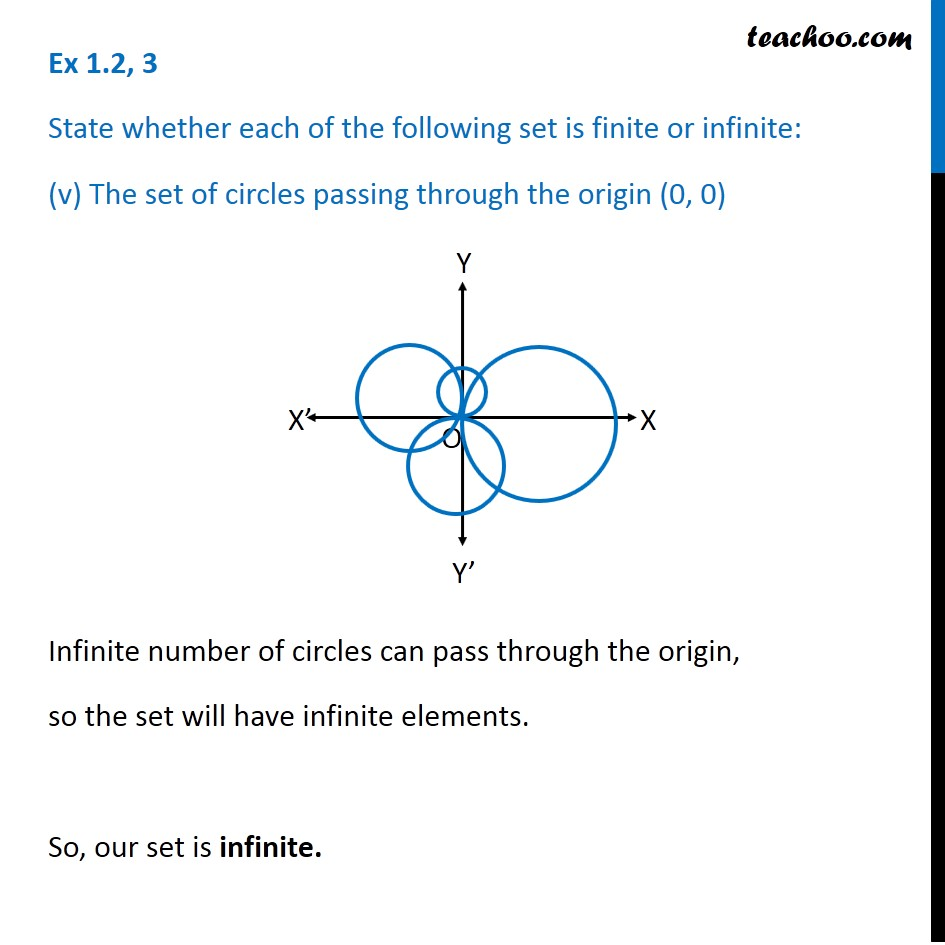1. Chapter 1 Class 11 Sets
2. Concept wise
3. Finite/Infinite

Transcript

Ex 1.2, 3 State whether each of the following set is finite or infinite: (i) The set of lines which are parallel to the x-axis There are infinite lines parallel to x- axis, so the set will have infinite elements. So, our set is infinite. Ex 1.2, 3 State whether each of the following set is finite or infinite: (ii) The set of letters in the English alphabet There are 26 letters in English alphabet, Therefore the set will have 26 elements. ∴ The set is finite. Ex 1.2, 3 State whether each of the following set is finite or infinite: (iii) The set of numbers which are multiple of 5 Multiples of 5 are 5, 10, 15, 20, 25, 30, 35, 40…… Multiples of 5 are infinite in number, So the set will have infinite elements. ∴ The set is infinite Ex 1.2, 3 State whether each of the following set is finite or infinite: (iv) The set of animals living on the earth The number of animals living on the earth is finite as the number of animals is countable. ∴ The set is finite. Ex 1.2, 3 State whether each of the following set is finite or infinite: (v) The set of circles passing through the origin (0, 0) Infinite number of circles can pass through the origin, so the set will have infinite elements. So, our set is infinite.

Finite/Infinite